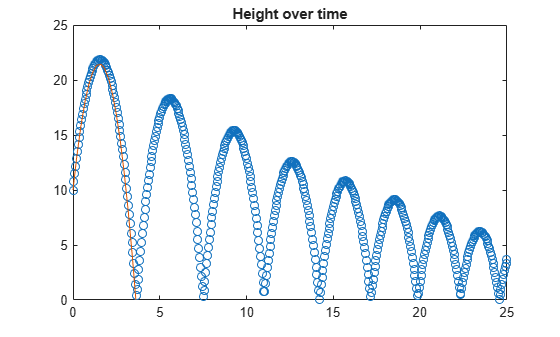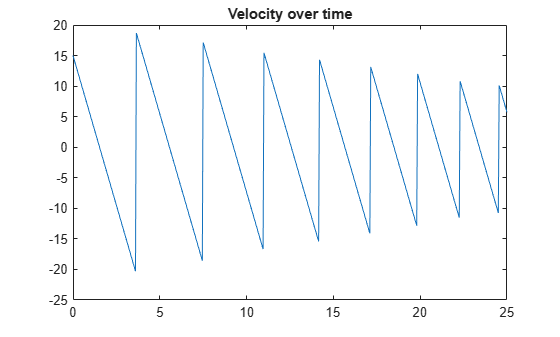Documentation

## Validate Simulink Model Using Symbolic Math Toolbox

This example shows how to model a bouncing ball, which is a classical hybrid dynamic system. This model includes both continuous dynamics and discrete transitions. It uses the Symbolic Math Toolbox to help explain some of the theory behind ODE solving in the Simulink® Model of a Bouncing Ball.

### Assumptions

• The ball rebounds with no angle

• There is no drag

• Height at time t=0 is 10 m

• Thrown with upwards velocity of 15 m/s

### Derivation

The coefficient of restitution is defined as

`${C}_{r}={v}_{b}-{v}_{a}/{u}_{a}-{u}_{b}$`

where v is the speed of object before impact and u is the speed after.We split the second order differential equation

`$\frac{{d}^{2}h}{d{t}^{2}}=-g$`

into

$\frac{dh}{dt}=v$ discretized as $\frac{h\left(t+\Delta t\right)-h\left(t\right)}{\Delta t}=v\left(t\right)$

and

$\frac{dv}{dt}=-g$ discretized as $\frac{v\left(t+\Delta t\right)-v\left(t\right)}{\Delta t}=-g$

We will use basic 1st order numerical integration using forward Euler.

`$h\left(t+\Delta t\right)=h\left(t\right)+v\left(t\right)\Delta t$`

`$v\left(t+\Delta t\right)=v\left(t\right)-g\Delta t$`

### Solve the Problem Analytically

Using the Symbolic Math Toolbox, we can approach the problem analytically. This allows us to solve certain problems more easily, such as determining precisely when the ball first hits the ground (below).

Declare our symbolic variables.

`syms g t H(t) h_0 v_0`

Split the second order differential equation $\frac{{d}^{2}h}{d{t}^{2}}=-g$into $\frac{dh}{dt}=v$ and $\frac{dv}{dt}=-g$.

```Dh = diff(H); D2h = diff(H, 2) == g```
```D2h(t) =  ```

Solve the ODE using `dsolve`:

`eqn = dsolve(D2h, H(0) == h_0, Dh(0) == v_0)`
```eqn =  $\frac{g {t}^{2}}{2}+{v}_{0} t+{h}_{0}$```

Parametrically explore the parabolic profile of motion using `subs`:

`eqn = subs(eqn, [h_0, v_0, g], [10, 15, -9.81])`
```eqn =  $-\frac{981 {t}^{2}}{200}+15 t+10$```

Find the time at which the ball hits the ground by solving for zero:

```assume(t > 0) tHit = solve(eqn == 0)```
```tHit =  $\frac{20 \sqrt{5} \sqrt{26}}{109}+\frac{500}{327}$```

Visualize the solution

```fplot(eqn,[0 10]) ylim([0 25])```Format your exact results using variable precision arithmetic with `vpa`:

`disp(['The ball with an initial height of 10m and velocity of 15m/s will hit the ground at ' char(vpa(tHit, 4)) ' seconds.'])`
```The ball with an initial height of 10m and velocity of 15m/s will hit the ground at 3.621 seconds. ```

### Solve the Problem Numerically

Setup Simulation Parameters

Properties of the ball

`c_bounce = .9; % Bouncing's coefficient of restitution; perfect restitution would be 1`

Properties of the simulation

```gravity = 9.8; % Gravity's acceleration (m/s) height_0 = 10; % Initial height at time t=0 (m) velocity_0=15; % Initial velocity at time t=0 (m/s)```

Declaring the simulation timestep

```dt = 0.05; % Animation timestep (s) t_final = 25; % Simulate period (s) t = 0:dt:t_final; % Timespan N = length(t); % Number of iterations```

Initialize simulation quantities

```h=[]; % Height of the ball as a function of time (m) v=[]; % Velocity of the ball (m/sec) as a function of time (m/s) h(1)=height_0; v(1)=velocity_0;```

Simulate the bouncing ball (we will use basic 1st order numerical integration using forward Euler):

```for i=1:N-1 v(i+1)=v(i)-gravity*dt; h(i+1)=h(i)+v(i)*dt; % When the ball bounces (the height is less than 0), % reverse the velocity and recalculate the position. % Using the coefficient of restitution if h(i+1) < 0 v(i)=-v(i)*c_bounce; v(i+1)=v(i)-gravity*dt; h(i+1)=0+v(i)*dt; end end```

Visualize and validate the simulation

```plot(t,h,'o') hold on fplot(eqn,[0 10]) title('Height over time') ylim([0 25]) hold off``````plot(t,v) title('Velocity over time')```### Compare your analytical results with your numeric results.

As a reminder the time of impact was:

`disp(['The ball with an initial height of 10m and velocity of 15m/s will hit the ground at ' char(vpa(tHit, 4)) ' seconds.'])`
```The ball with an initial height of 10m and velocity of 15m/s will hit the ground at 3.621 seconds. ```

From the numerical simulation we can find the closest value in the simulation when $\mathit{h}\left({\mathit{t}}_{\mathit{i}}\right)\approx 0$

```i = ceil(double(tHit/dt)); t([i-1 i i+1]) ```
```ans = 1×3 3.5500 3.6000 3.6500 ```
```plot(t,h,'o') hold on fplot(eqn,[0 10]) plot(t([i-1 i i+1 ]),h([i-1 i i+1 ]),'*R') title('Height over time') xlim([0 5]) ylim([0 25]) hold off```#### Mathematical Modeling with Symbolic Math Toolbox

Get examples and videos